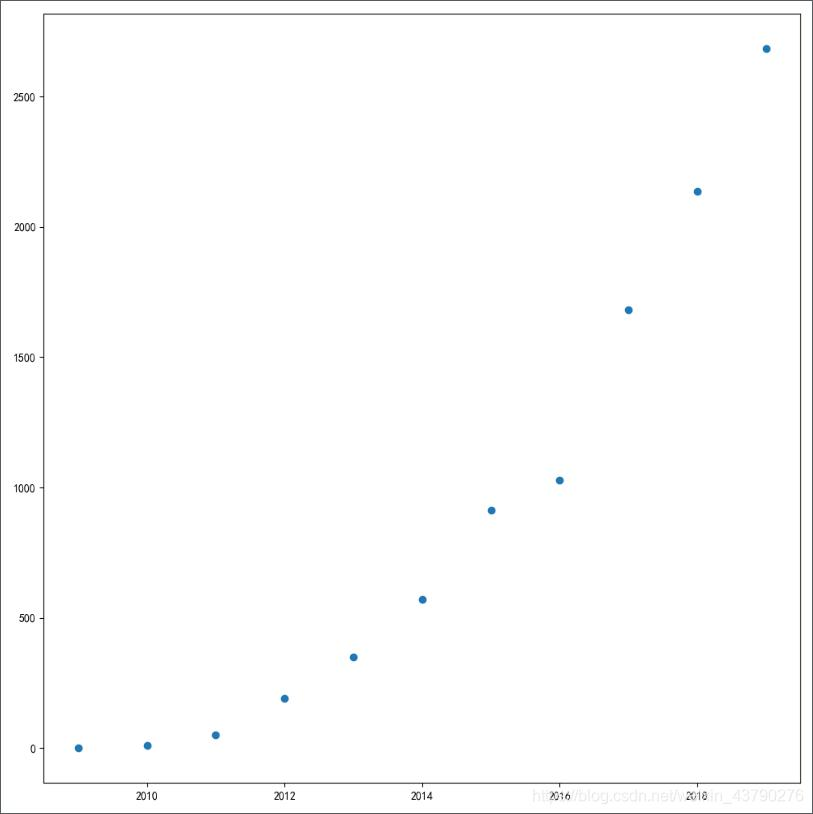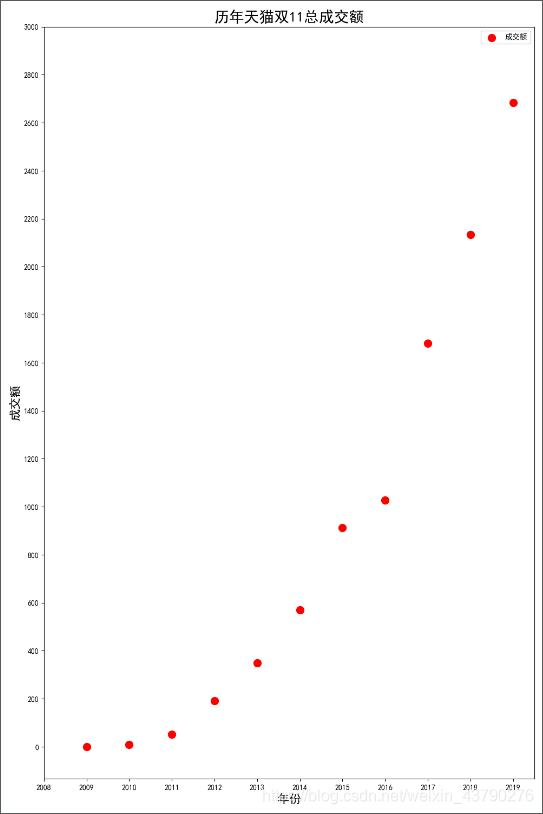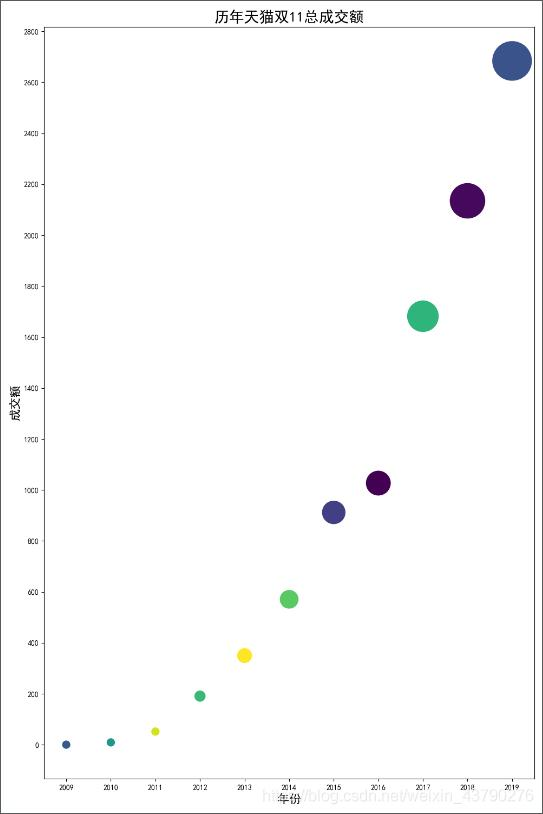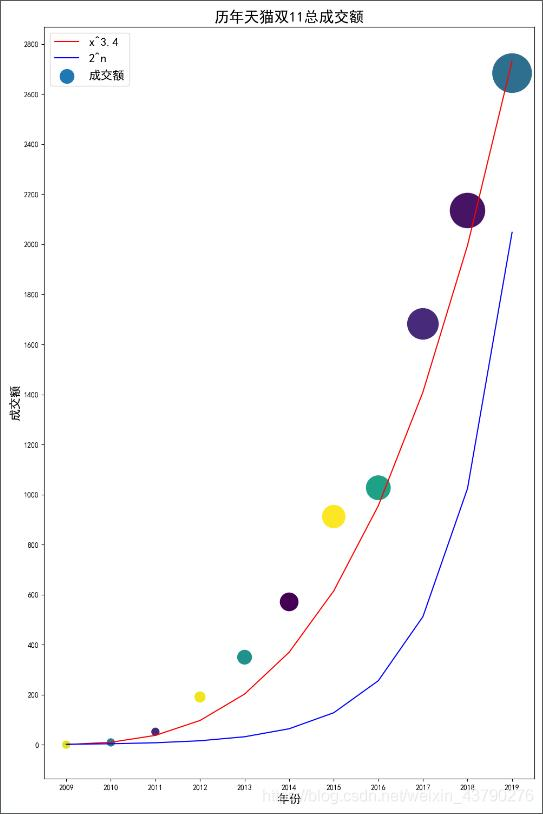• 本代码是利用python绘制散点图，使用xlrd读取Excel文件，
• 前言上篇文章介绍了使用matplotlib绘制折线图，参考：...一、matplotlib绘制散点图# coding=utf-8import matplotlib.pyplot as pltyears = [2009, 2010, 2011, 2012, 2013, 201...
前言上篇文章介绍了使用matplotlib绘制折线图，参考：https://www.jb51.net/article/198991.htm，本篇文章继续介绍使用matplotlib绘制散点图。一、matplotlib绘制散点图# coding=utf-8import matplotlib.pyplot as pltyears = [2009, 2010, 2011, 2012, 2013, 2014, 2015, 2016, 2017, 2018, 2019]turnovers = [0.5, 9.36, 52, 191, 350, 571, 912, 1027, 1682, 2135, 2684]plt.figure(figsize=(10, 10), dpi=100)plt.scatter(years, turnovers)plt.show()运行结果：scatter(): matplotlib中绘制散点图的函数。可以传入很多参数，一般传入两个列表，分别是散点图中的x值和y值。上面的例子中使用2009年至2019年这十一年天猫双11的总成交额数据。散点图根据提供的两组数据，构成图形中的多个坐标点。根据坐标点的分布，分析两个变量之间是否存在某种关联，或总结坐标点的分布趋势，用于预测数据的走势。上面的代码已经实现了简单的散点图，但只把点绘制出来了，很多信息都不完整，所以需要进行优化。二、matplotlib优化散点图import matplotlib.pyplot as pltyears = [2009, 2010, 2011, 2012, 2013, 2014, 2015, 2016, 2017, 2018, 2019]turnovers = [0.5, 9.36, 52, 191, 350, 571, 912, 1027, 1682, 2135, 2684]plt.figure(figsize=(10, 15), dpi=100)plt.scatter(years, turnovers, c='red', s=100, label='成交额')plt.xticks(range(2008, 2020, 1))plt.yticks(range(0, 3200, 200))plt.xlabel("年份", fontdict={'size': 16})plt.ylabel("成交额", fontdict={'size': 16})plt.title("历年天猫双11总成交额", fontdict={'size': 20})plt.legend(loc='best')plt.show()运行结果：在第一次绘制的散点图中，已经看出了点的大概分布情况，所以在使用figure()函数创建图像时，可以修改figsize参数调整图像尺寸，设置更好的图像比例。在调用scatter()函数绘制散点图时，使用c='颜色'来设置点的颜色，使用s='大小'来设置点的大小，并设置label用于图例展示。第一次的散点图中，x轴上没有显示所有的年份刻度，最后一个点已经分布到了图形的右上角，所以使用xticks()和yticks()来设置x轴和y轴的刻度标签和范围。使用xlabel()和ylabel()设置x轴和y轴的标签，说明x轴和y轴的含义。使用title()设置散点图的标题，说明散点图展示的数据。使用legend()将图例展示出来。这样一张基本功能完整，信息完整的散点图就完成了。三、matplotlib散点图区分点的颜色和大小import matplotlib.pyplot as pltimport numpy as npyears = [2009, 2010, 2011, 2012, 2013, 2014, 2015, 2016, 2017, 2018, 2019]turnovers = [0.5, 9.36, 52, 191, 350, 571, 912, 1027, 1682, 2135, 2684]plt.figure(figsize=(10, 15), dpi=100)size = list()for tur in turnovers:size.append(tur) if tur > 100 else size.append(100)plt.xticks(range(2008, 2020, 1))plt.yticks(range(0, 3200, 200))plt.scatter(years, turnovers, c=np.random.randint(0, 50, 11), s=size)plt.xlabel("年份", fontdict={'size': 16})plt.ylabel("成交额", fontdict={'size': 16})plt.title("历年天猫双11总成交额", fontdict={'size': 20})plt.show()运行结果：上一张散点图中已经对数据作了基本的展示，为了使数据展示效果更好，可以对散点图进行美化。数据是历年双11的总成交额，每年的数据是独立的，可以用不同的颜色来区分。这里使用numpy中的random.randint()随机生成11个值，将这11个随机的值传给scatter()函数中的c参数，使每一个点的颜色不一样，可以更好地表示每个点的独立性。pip install numpy -i https://pypi.tuna.tsinghua.edu.cn/simple成交额是逐年变化的，为了从散点图中体现出大小的差异，可以根据成交额的大小设置点的大小。这里直接将成交额作为点的大小(成交额很小的设置一个值，图形中的点不小于这个值)，得到由11个值组成的列表，传给scatter()函数中的s参数，可以体现每个点的大小差异(成交额越大点越大)。四、matplotlib散点图的趋势简单分析import matplotlib.pyplot as pltimport numpy as npimport mathyears = [2009, 2010, 2011, 2012, 2013, 2014, 2015, 2016, 2017, 2018, 2019]turnovers = [0.5, 9.36, 52, 191, 350, 571, 912, 1027, 1682, 2135, 2684]squares = [math.pow(year-2008, 3.3) for year in years]powers = [math.pow(2, year-2008) for year in years]plt.figure(figsize=(10, 15), dpi=100)size = list()for tur in turnovers:size.append(tur) if tur > 100 else size.append(100)plt.xticks(range(2008, 2020, 1))plt.yticks(range(0, 3200, 200))plt.scatter(years, turnovers, c=np.random.randint(0, 50, 11), s=size, label='成交额')plt.plot(years, squares, color='red', label='x^3.4')plt.plot(years, powers, color='blue', label='2^n')plt.legend(loc='best', fontsize=16, markerscale=0.5)plt.xlabel("年份", fontdict={'size': 16})plt.ylabel("成交额", fontdict={'size': 16})plt.title("历年天猫双11总成交额", fontdict={'size': 20})plt.show()运行结果：散点图的作用主要是用于分析数据的趋势，用于预测未来的数据。比如我想预测2020年天猫双11的总成交额，通过对比的方式，简单分析一下这个趋势更接近指数函数还是更接近多次函数。在散点图中，我绘制了两条曲线，y=2^x和y=x^(3.4)，一条是2为底的指数函数，一条是x的3.4次方(三次函数ax^3+bx^2+cx+d)，可以看到双11总成交额的变化趋势更接近三次函数。这里我只是简单对比一下，三次函数还有二次项、一次项和常数项，所以x^(3.4)中的0.4可以通过二次项、一次项和常数项来补充，指数函数的变化趋势太快，与双11总成交额的变化趋势差异很大。这种简单对比是很粗糙的，只是为了说明散点图可以用于分析趋势。真实的分析不能简单看每年的数据，需要考虑很多因素(甚至因为某个因素的加入，成交额已经快到天花板了，很可能后面会下降)。总结到此这篇关于Python利用matplotlib绘制散点图的文章就介绍到这了,更多相关Python matplotlib绘制散点图内容请搜索python博客以前的文章或继续浏览下面的相关文章希望大家以后多多支持python博客！
展开全文• 前言S7k免费资源网上篇文章介绍了使用matplotlib绘制折线图，参考：...S7k免费资源网一、matplotlib绘制散点图S7k免费资源网# coding=utf-8import matplotlib.pyplot as pltyears = [2009, 201...
前言S7k免费资源网上篇文章介绍了使用matplotlib绘制折线图，参考：https://www.jb51.net/article/198991.htm，本篇文章继续介绍使用matplotlib绘制散点图。S7k免费资源网一、matplotlib绘制散点图S7k免费资源网# coding=utf-8import matplotlib.pyplot as pltyears = [2009, 2010, 2011, 2012, 2013, 2014, 2015, 2016, 2017, 2018, 2019]turnovers = [0.5, 9.36, 52, 191, 350, 571, 912, 1027, 1682, 2135, 2684]plt.figure(figsize=(10, 10), dpi=100)plt.scatter(years, turnovers)plt.show()运行结果：S7k免费资源网S7k免费资源网scatter(): matplotlib中绘制散点图的函数。可以传入很多参数，一般传入两个列表，分别是散点图中的x值和y值。上面的例子中使用2009年至2019年这十一年天猫双11的总成交额数据。S7k免费资源网散点图根据提供的两组数据，构成图形中的多个坐标点。根据坐标点的分布，分析两个变量之间是否存在某种关联，或总结坐标点的分布趋势，用于预测数据的走势。S7k免费资源网上面的代码已经实现了简单的散点图，但只把点绘制出来了，很多信息都不完整，所以需要进行优化。S7k免费资源网二、matplotlib优化散点图S7k免费资源网import matplotlib.pyplot as pltyears = [2009, 2010, 2011, 2012, 2013, 2014, 2015, 2016, 2017, 2018, 2019]turnovers = [0.5, 9.36, 52, 191, 350, 571, 912, 1027, 1682, 2135, 2684]plt.figure(figsize=(10, 15), dpi=100)plt.scatter(years, turnovers, c='red', s=100, label='成交额')plt.xticks(range(2008, 2020, 1))plt.yticks(range(0, 3200, 200))plt.xlabel("年份", fontdict={'size': 16})plt.ylabel("成交额", fontdict={'size': 16})plt.title("历年天猫双11总成交额", fontdict={'size': 20})plt.legend(loc='best')plt.show()运行结果：S7k免费资源网S7k免费资源网在第一次绘制的散点图中，已经看出了点的大概分布情况，所以在使用figure()函数创建图像时，可以修改figsize参数调整图像尺寸，设置更好的图像比例。S7k免费资源网在调用scatter()函数绘制散点图时，使用c='颜色'来设置点的颜色，使用s='大小'来设置点的大小，并设置label用于图例展示。S7k免费资源网第一次的散点图中，x轴上没有显示所有的年份刻度，最后一个点已经分布到了图形的右上角，所以使用xticks()和yticks()来设置x轴和y轴的刻度标签和范围。S7k免费资源网使用xlabel()和ylabel()设置x轴和y轴的标签，说明x轴和y轴的含义。使用title()设置散点图的标题，说明散点图展示的数据。使用legend()将图例展示出来。S7k免费资源网这样一张基本功能完整，信息完整的散点图就完成了。S7k免费资源网三、matplotlib散点图区分点的颜色和大小S7k免费资源网import matplotlib.pyplot as pltimport numpy as npyears = [2009, 2010, 2011, 2012, 2013, 2014, 2015, 2016, 2017, 2018, 2019]turnovers = [0.5, 9.36, 52, 191, 350, 571, 912, 1027, 1682, 2135, 2684]plt.figure(figsize=(10, 15), dpi=100)size = list()for tur in turnovers:size.append(tur) if tur > 100 else size.append(100)plt.xticks(range(2008, 2020, 1))plt.yticks(range(0, 3200, 200))plt.scatter(years, turnovers, c=np.random.randint(0, 50, 11), s=size)plt.xlabel("年份", fontdict={'size': 16})plt.ylabel("成交额", fontdict={'size': 16})plt.title("历年天猫双11总成交额", fontdict={'size': 20})plt.show()运行结果：S7k免费资源网S7k免费资源网上一张散点图中已经对数据作了基本的展示，为了使数据展示效果更好，可以对散点图进行美化。S7k免费资源网数据是历年双11的总成交额，每年的数据是独立的，可以用不同的颜色来区分。这里使用numpy中的random.randint()随机生成11个值，将这11个随机的值传给scatter()函数中的c参数，使每一个点的颜色不一样，可以更好地表示每个点的独立性。S7k免费资源网pip install numpy -i https://pypi.tuna.tsinghua.edu.cn/simple成交额是逐年变化的，为了从散点图中体现出大小的差异，可以根据成交额的大小设置点的大小。这里直接将成交额作为点的大小(成交额很小的设置一个值，图形中的点不小于这个值)，得到由11个值组成的列表，传给scatter()函数中的s参数，可以体现每个点的大小差异(成交额越大点越大)。S7k免费资源网四、matplotlib散点图的趋势简单分析S7k免费资源网import matplotlib.pyplot as pltimport numpy as npimport mathyears = [2009, 2010, 2011, 2012, 2013, 2014, 2015, 2016, 2017, 2018, 2019]turnovers = [0.5, 9.36, 52, 191, 350, 571, 912, 1027, 1682, 2135, 2684]squares = [math.pow(year-2008, 3.3) for year in years]powers = [math.pow(2, year-2008) for year in years]plt.figure(figsize=(10, 15), dpi=100)size = list()for tur in turnovers:size.append(tur) if tur > 100 else size.append(100)plt.xticks(range(2008, 2020, 1))plt.yticks(range(0, 3200, 200))plt.scatter(years, turnovers, c=np.random.randint(0, 50, 11), s=size, label='成交额')plt.plot(years, squares, color='red', label='x^3.4')plt.plot(years, powers, color='blue', label='2^n')plt.legend(loc='best', fontsize=16, markerscale=0.5)plt.xlabel("年份", fontdict={'size': 16})plt.ylabel("成交额", fontdict={'size': 16})plt.title("历年天猫双11总成交额", fontdict={'size': 20})plt.show()运行结果：S7k免费资源网S7k免费资源网散点图的作用主要是用于分析数据的趋势，用于预测未来的数据。比如我想预测2020年天猫双11的总成交额，通过对比的方式，简单分析一下这个趋势更接近指数函数还是更接近多次函数。S7k免费资源网在散点图中，我绘制了两条曲线，y=2^x和y=x^(3.4)，一条是2为底的指数函数，一条是x的3.4次方(三次函数ax^3+bx^2+cx+d)，可以看到双11总成交额的变化趋势更接近三次函数。S7k免费资源网这里我只是简单对比一下，三次函数还有二次项、一次项和常数项，所以x^(3.4)中的0.4可以通过二次项、一次项和常数项来补充，指数函数的变化趋势太快，与双11总成交额的变化趋势差异很大。这种简单对比是很粗糙的，只是为了说明散点图可以用于分析趋势。真实的分析不能简单看每年的数据，需要考虑很多因素(甚至因为某个因素的加入，成交额已经快到天花板了，很可能后面会下降)。S7k免费资源网总结S7k免费资源网
展开全文• 前言上篇文章介绍了使用matplotlib绘制折线图，参考：...一、matplotlib绘制散点图# coding=utf-8import matplotlib.pyplot as pltyears = [2009, 2010, 2011, 2012, 2013, 201...
前言上篇文章介绍了使用matplotlib绘制折线图，参考：https://www.jb51.net/article/198991.htm，本篇文章继续介绍使用matplotlib绘制散点图。一、matplotlib绘制散点图# coding=utf-8import matplotlib.pyplot as pltyears = [2009, 2010, 2011, 2012, 2013, 2014, 2015, 2016, 2017, 2018, 2019]turnovers = [0.5, 9.36, 52, 191, 350, 571, 912, 1027, 1682, 2135, 2684]plt.figure(figsize=(10, 10), dpi=100)plt.scatter(years, turnovers)plt.show()运行结果：scatter(): matplotlib中绘制散点图的函数。可以传入很多参数，一般传入两个列表，分别是散点图中的x值和y值。上面的例子中使用2009年至2019年这十一年天猫双11的总成交额数据。散点图根据提供的两组数据，构成图形中的多个坐标点。根据坐标点的分布，分析两个变量之间是否存在某种关联，或总结坐标点的分布趋势，用于预测数据的走势。上面的代码已经实现了简单的散点图，但只把点绘制出来了，很多信息都不完整，所以需要进行优化。二、matplotlib优化散点图import matplotlib.pyplot as pltyears = [2009, 2010, 2011, 2012, 2013, 2014, 2015, 2016, 2017, 2018, 2019]turnovers = [0.5, 9.36, 52, 191, 350, 571, 912, 1027, 1682, 2135, 2684]plt.figure(figsize=(10, 15), dpi=100)plt.scatter(years, turnovers, c='red', s=100, label='成交额')plt.xticks(range(2008, 2020, 1))plt.yticks(range(0, 3200, 200))plt.xlabel("年份", fontdict={'size': 16})plt.ylabel("成交额", fontdict={'size': 16})plt.title("历年天猫双11总成交额", fontdict={'size': 20})plt.legend(loc='best')plt.show()运行结果：在第一次绘制的散点图中，已经看出了点的大概分布情况，所以在使用figure()函数创建图像时，可以修改figsize参数调整图像尺寸，设置更好的图像比例。在调用scatter()函数绘制散点图时，使用c='颜色'来设置点的颜色，使用s='大小'来设置点的大小，并设置label用于图例展示。第一次的散点图中，x轴上没有显示所有的年份刻度，最后一个点已经分布到了图形的右上角，所以使用xticks()和yticks()来设置x轴和y轴的刻度标签和范围。使用xlabel()和ylabel()设置x轴和y轴的标签，说明x轴和y轴的含义。使用title()设置散点图的标题，说明散点图展示的数据。使用legend()将图例展示出来。这样一张基本功能完整，信息完整的散点图就完成了。三、matplotlib散点图区分点的颜色和大小import matplotlib.pyplot as pltimport numpy as npyears = [2009, 2010, 2011, 2012, 2013, 2014, 2015, 2016, 2017, 2018, 2019]turnovers = [0.5, 9.36, 52, 191, 350, 571, 912, 1027, 1682, 2135, 2684]plt.figure(figsize=(10, 15), dpi=100)size = list()for tur in turnovers:size.append(tur) if tur > 100 else size.append(100)plt.xticks(range(2008, 2020, 1))plt.yticks(range(0, 3200, 200))plt.scatter(years, turnovers, c=np.random.randint(0, 50, 11), s=size)plt.xlabel("年份", fontdict={'size': 16})plt.ylabel("成交额", fontdict={'size': 16})plt.title("历年天猫双11总成交额", fontdict={'size': 20})plt.show()运行结果：上一张散点图中已经对数据作了基本的展示，为了使数据展示效果更好，可以对散点图进行美化。数据是历年双11的总成交额，每年的数据是独立的，可以用不同的颜色来区分。这里使用numpy中的random.randint()随机生成11个值，将这11个随机的值传给scatter()函数中的c参数，使每一个点的颜色不一样，可以更好地表示每个点的独立性。pip install numpy -i https://pypi.tuna.tsinghua.edu.cn/simple成交额是逐年变化的，为了从散点图中体现出大小的差异，可以根据成交额的大小设置点的大小。这里直接将成交额作为点的大小(成交额很小的设置一个值，图形中的点不小于这个值)，得到由11个值组成的列表，传给scatter()函数中的s参数，可以体现每个点的大小差异(成交额越大点越大)。四、matplotlib散点图的趋势简单分析import matplotlib.pyplot as pltimport numpy as npimport mathyears = [2009, 2010, 2011, 2012, 2013, 2014, 2015, 2016, 2017, 2018, 2019]turnovers = [0.5, 9.36, 52, 191, 350, 571, 912, 1027, 1682, 2135, 2684]squares = [math.pow(year-2008, 3.3) for year in years]powers = [math.pow(2, year-2008) for year in years]plt.figure(figsize=(10, 15), dpi=100)size = list()for tur in turnovers:size.append(tur) if tur > 100 else size.append(100)plt.xticks(range(2008, 2020, 1))plt.yticks(range(0, 3200, 200))plt.scatter(years, turnovers, c=np.random.randint(0, 50, 11), s=size, label='成交额')plt.plot(years, squares, color='red', label='x^3.4')plt.plot(years, powers, color='blue', label='2^n')plt.legend(loc='best', fontsize=16, markerscale=0.5)plt.xlabel("年份", fontdict={'size': 16})plt.ylabel("成交额", fontdict={'size': 16})plt.title("历年天猫双11总成交额", fontdict={'size': 20})plt.show()运行结果：散点图的作用主要是用于分析数据的趋势，用于预测未来的数据。比如我想预测2020年天猫双11的总成交额，通过对比的方式，简单分析一下这个趋势更接近指数函数还是更接近多次函数。在散点图中，我绘制了两条曲线，y=2^x和y=x^(3.4)，一条是2为底的指数函数，一条是x的3.4次方(三次函数ax^3+bx^2+cx+d)，可以看到双11总成交额的变化趋势更接近三次函数。这里我只是简单对比一下，三次函数还有二次项、一次项和常数项，所以x^(3.4)中的0.4可以通过二次项、一次项和常数项来补充，指数函数的变化趋势太快，与双11总成交额的变化趋势差异很大。这种简单对比是很粗糙的，只是为了说明散点图可以用于分析趋势。真实的分析不能简单看每年的数据，需要考虑很多因素(甚至因为某个因素的加入，成交额已经快到天花板了，很可能后面会下降)。总结到此这篇关于Python利用matplotlib绘制散点图的文章就介绍到这了,更多相关Python matplotlib绘制散点图内容请搜索python博客以前的文章或继续浏览下面的相关文章希望大家以后多多支持python博客！
展开全文• 一、matplotlib绘制散点图 # coding=utf-8 import matplotlib.pyplot as plt years = [2009, 2010, 2011, 2012, 2013, 2014, 2015, 2016, 2017, 2018, 2019] turnovers = [0.5, 9.36, 52, 191, 350, 571, 912, 1027...
• // Import necessary packages from matplotlib import pyplot as...//定义一个函数实现利用文档中某几列数据生成散点图 def plotData(x1,y1,x2,y2): plt.figure(figsize=(8,8))#设置图片大小 plt.scatter(x1,...
// Import necessary packages
from matplotlib import pyplot as plt
import numpy as np
//定义一个函数实现利用文档中某几列数据生成散点图
def plotData(x1,y1,x2,y2):
plt.figure(figsize=(8,8))#设置图片大小
plt.scatter(x1,y1,s=30,c=‘red’,marker=‘o’,alpha=1,label=‘gound truth’)#设置
plt.scatter(x2,y2,s=30,c=‘blue’,marker=‘o’,alpha=1,label=‘predicted’)
plt.title('error ')#图片标题
plt.xlabel(‘X(m)’)#X轴名称
plt.ylabel(‘Y(m)’)
plt.legend(loc=‘upper right’)#标签
plt.savefig(“examples.jpg”)
plt.show()
//读取文档中需要的某几列数据
x1 = []
y1 = []
x2 = []
y2 = []
x1 = [float(l.split()) for l in open(“aaa.txt”)]
y1 = [float(l.split()) for l in open(“aaa.txt”)]
x2 = [float(l.split()) for l in open(“aaa.txt”)]
y2 = [float(l.split()) for l in open(“aaa.txt”)]
plotData(x1,y1,x2,y2)


展开全文• 散点图是指在数理统计回归分析中，数据点在直角坐标系平面上的分布图，散点图表示因变量随自变量而变化的大致趋势，由此趋势可以选择合适的函数进行经验分布的拟合，进而找到变量之间的函数关系。 二、散点图有什么...
• 2,3,4,5], [1,2,3,4,5], s=30, c="b", marker="o", label="Admitted") 接着对坐标系对象调用scatter()方法设置坐标轴，前两个参数为长度相同的列表，表示平面点的x，y轴坐标值，参数s表示绘制点的大小，参数c表示...
• 本项目是利用五年左右的世界地震数据，通过python的pandas库、matplotlib库、basemap库等进行数据可视化，绘制出地震散点图。主要代码如下所示 from __future__ import division import pandas as pd from pandas ...
• 遇到了一个气象站实际观测数据与预测数据之间的对比，因此考虑绘制一个散点图来进行展示，但是我的实测数据与预测数据统计下来有100多万个样本点(时间分辨率比较高、统计时间较长)，起初绘制散点图是这样的。...
• 本项目是利用五年左右的世界地震数据，通过python的pandas库、matplotlib库、basemap库等进行数据可视化，绘制出地震散点图。主要代码如下所示from __future__ import divisionimport pandas as pdfrom pandas ...
• python 绘制中国地图并利用经纬度标注散点 所需要的包：GeoPandas，安装教程有很多，自行百度即可。 用到的中国地级市shp文件：链接：https://pan.baidu.com/s/18aaxczrz4tIRMeCusOrDQA 提取码：rav1 一、...
• 上篇博客已经讲述了如何绘制折线图，现在来学习绘制散点图，直方图和条形图 1、散点图 绘制三月份和十月份的温度变化情况 from matplotlib import pyplot as plt import random from matplotlib import font_manager...数据可视化 可视化
• 本项目是利用五年左右的世界地震数据，通过python的pandas库、matplotlib库、basemap库等进行数据可视化，绘制出地震散点图。主要代码如下所示from __future__ import divisionimport pandas as pdfrom pandas ...
• 1、scatter函数原型2、其中散点的形状参数marker如下：3、其中颜色参数c如下:4、基本的使用方法如下： [python] view plain copy #导入必要的模块  import numpy as np  import matplotlib.pyplot as p
• 如果你想做很多个散点图，却又不想使用Excel一个一个地画，一张一张图调整。那么你可以考虑使用Python。首先，需要安装并启动anaconda3，过程略。开始写代码了。01基本库的导入，以及为避免后续问题和提前做的设定，...
• random_walk.py文件，一个类用于生成散点图     from random import choice class RandomWalk():  """"一个生成随机漫步的属性"""    def __init__(self,num_points...
• 遇到了一个气象站实际观测数据与预测数据之间的对比，因此考虑绘制一个散点图来进行展示，但是我的实测数据与预测数据统计下来有100多万个样本点(时间分辨率比较高、统计时间较长)，起初绘制散点图是这样的。...
• 怎样用Python绘制散点图？本文逐一为你解答。作者：屈希峰来源：华章科技01 概述散点图(Scatter)又称散点分布图，是以一个变量为横坐标，另一个变量为纵坐标，利用散点(坐标点)的分布形态反映变量统计关系的一种图形...python散点图
• import pandas as pd import numpy as np import matplotlib.pyplot as plt import random N=50 x=np.random.randn(N) y=np.random.randn(N) plt.scatter(x,y,s=50,c='b',marker='o',alpha=0.5) ...
• 遇到了一个气象站实际观测数据与预测数据之间的对比，因此考虑绘制一个散点图来进行展示，但是我的实测数据与预测数据统计下来有100多万个样本点(时间分辨率比较高、统计时间较长)，起初绘制散点图是这样的。......

# 利用python绘制散点图python 订阅